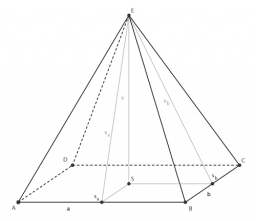In a regular quadrilateral pyramid, the length of the base edge is a = 8 cm, and the length of the side edge is h = 17 cm. Calculate the surface of the pyramid.

S =  328.3634 cm2

### Step-by-step explanation:Did you find an error or inaccuracy? Feel free to write us. Thank you!

Tips to related online calculators
Pythagorean theorem is the base for the right triangle calculator.

#### You need to know the following knowledge to solve this word math problem:

We encourage you to watch this tutorial video on this math problem: